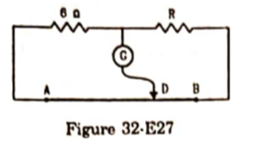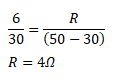# The potentiometer wire AB shown in figure (32-E27)

Question:

The potentiometer wire $A B$ shown in figure (32-E27) is $50 \mathrm{~cm}$ long. When $A D=30 \mathrm{~cm}$, no deflection occurs in the galvanometer. Find R.Solution: